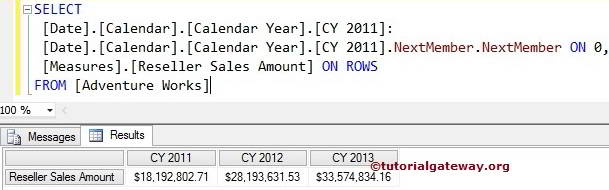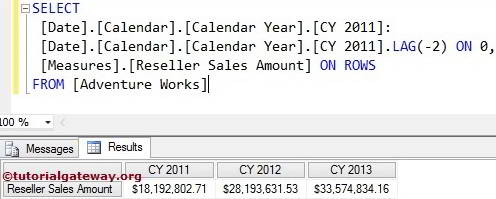# MDX NextMember Function

The MDX NextMember function will return the same level member at the Next position.

## MDX Nextmember Function Syntax

The basic syntax of the Nextmember Function in Multidimensional Expression is as shown below:

`Member_Expression.NEXTMEMBER`

Member_Expression: Any Multidimensional Expression that returns valid Member.

The Nextmember Function is used to move forward between Members at the same level. For example, If we mentioned City member as Member_Expression, the Nextmember function will move forward between remaining Cities. It will not go Level Up (State – Province) or Level down (Postal Code).

For this Nextmember function example, we are going to use below shown data.

## MDX Nextmember Function Example

In this example, we will show you, How to write the NextMember function in Multidimensional Expression query. The following query will return the Reseller Sales amount of the Calendar Year 2012 because [CY 2011].NEXTMEMBER will look for a year after 2011, which is 2012.

```SELECT [Date].[Calendar].[Calendar Year].[CY 2011].NextMember ON COLUMNS,
[Measures].[Reseller Sales Amount] ON ROWS

## MDX Nextmember Function Example 2

In this example, we will show how to write the multiple NextMember functions in a single Multidimensional Expression query. The following MDX query will return the Reseller Sales amount of the Calendar Year 2013.

```SELECT [Date].[Calendar].[Calendar Year].[CY 2011].NEXTMEMBER.NEXTMEMBER ON 0
,[Measures].[Reseller Sales Amount] ON ROWS

First, [CY 2011].NEXTMEMBER will look for year after 2011, which is 2012

`[Date].[Calendar].[Calendar Year].[CY 2011].NextMember`

Second, [CY 2011].Nextmember.Nextmember will look for year after 2012, which is 2013

`[Date].[Calendar].[Calendar Year].[CY 2011].NextMember.NextMember`

## Finding Range Using MDX Nextmember Function

How to find the Range using Nextmember function?. The following query will return the Reseller Sales amount from Calendar Year 2011 to 2013 because we used the range symbol (:) between [CY 2011] and [CY 2011].Nextmember.Nextmember.

```SELECT
[Date].[Calendar].[Calendar Year].[CY 2011]:
[Date].[Calendar].[Calendar Year].[CY 2011].NextMember.NextMember ON 0
,[Measures].[Reseller Sales Amount] ON ROWS## MDX Nextmember Function Alternative 1

In this example, We are going to use Lead Function with Positive integer value to achieve above results. Please refer MDX Lead Function article to understand the function

```SELECT
[Date].[Calendar].[Calendar Year].[CY 2011]:
,[Measures].[Reseller Sales Amount] ON ROWS## MDX Nextmember Function Alternative 2

In this example, we are going to use Lag Function with Negative integer value. Please refer MDX Lag Function article to understand the function

```SELECT
[Date].[Calendar].[Calendar Year].[CY 2011]:
[Date].[Calendar].[Calendar Year].[CY 2011].LAG(-2) ON 0
,[Measures].[Reseller Sales Amount] ON ROWS